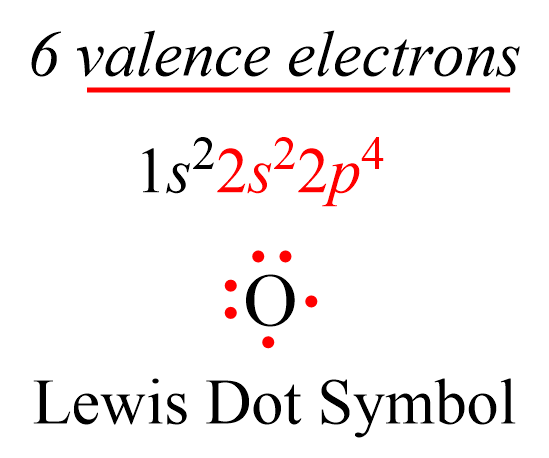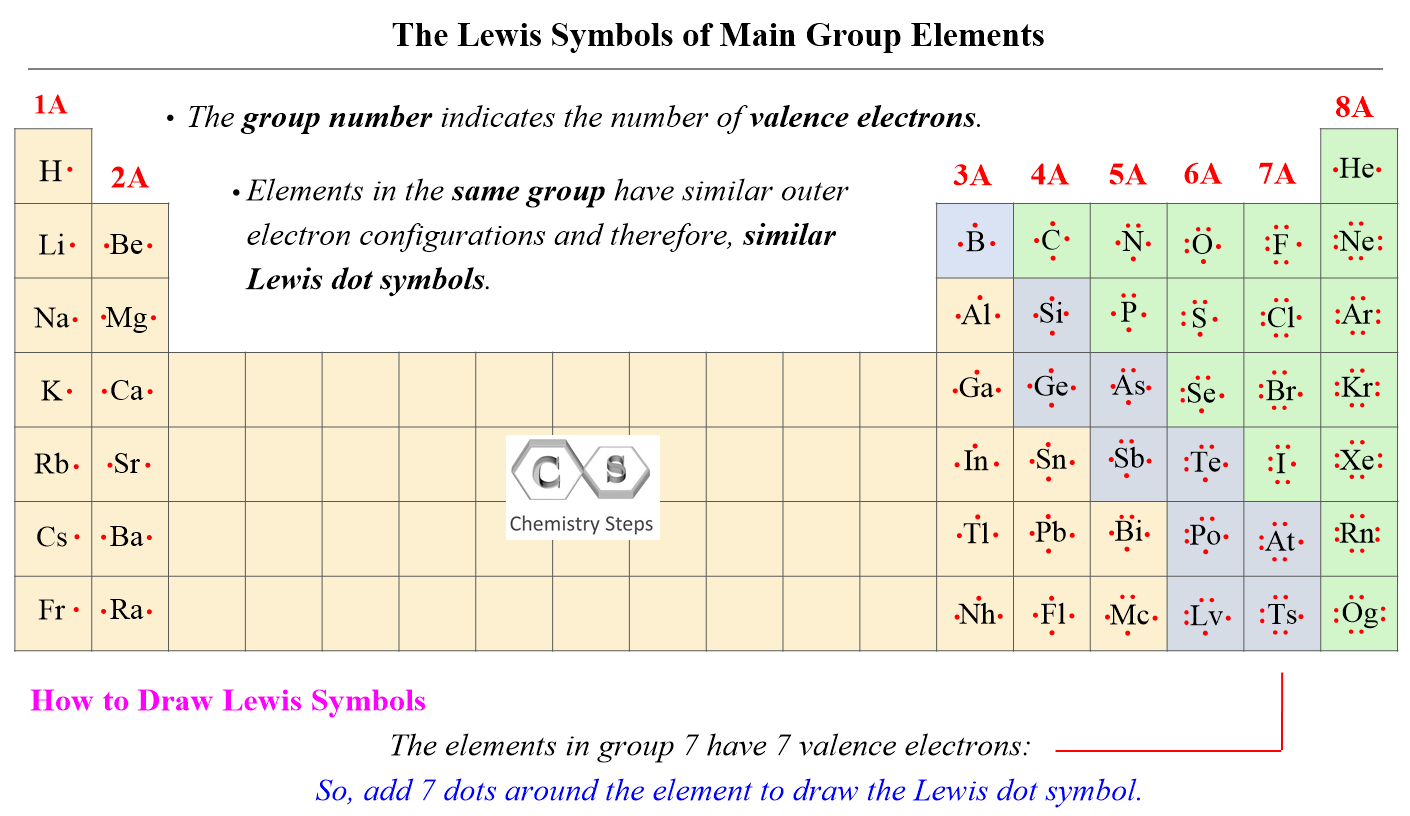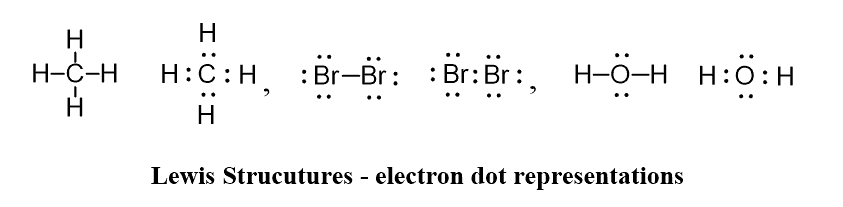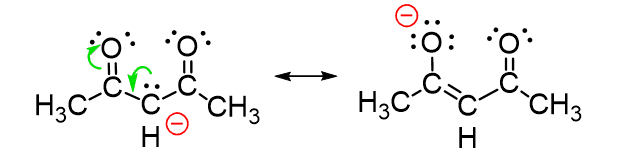## General Chemistry

Lewis dot symbols consist of the symbol of an element surrounded by its valence electrons represented as dots. So, to draw the Lewis dot symbol of an element, we must first know how many valence electrons it has. Remember, this is easily determined based on the group number of the element since valence electrons are the ones in the outermost energy shell of the element. For example, oxygen is in group 6, and therefore, it has six valence electrons, we can show the Lewis dot symbol as:And just like this, we can draw the Lewis symbols of all the main group elements. Simply add dots one by one to match the number of valence electrons (group number). For example, Mg is a Group 2A element and has two dots for two valence electrons, and C has four dots in the Lewis symbol since it is in Group 4A. If it is noted as 14 instead of 4A, you can subtract 10 from the group number and determine the valence electrons.Notice that the only exception when the number of valence electrons does not match the group number is helium. Transition metals, lanthanides, and actinides all have incomplete inner shells of electrons, and therefore, we cannot in general write simple Lewis dot symbols for them.

# Why Do We Need Lewis Symbols?

Lewis dot symbols show the number of valence electrons and therefore, they are the basis of understanding the principles of chemical bonding which originate by sharing these electrons between different atoms. These notations are needed to draw Lewis structures that demonstrate the connectivity of atoms in molecules as well as resonance structures which show the distribution of electrons on the molecule. Below are some examples of Lewis structures of simple and some larger organic molecules which we are going to discuss in the next article:Check Also